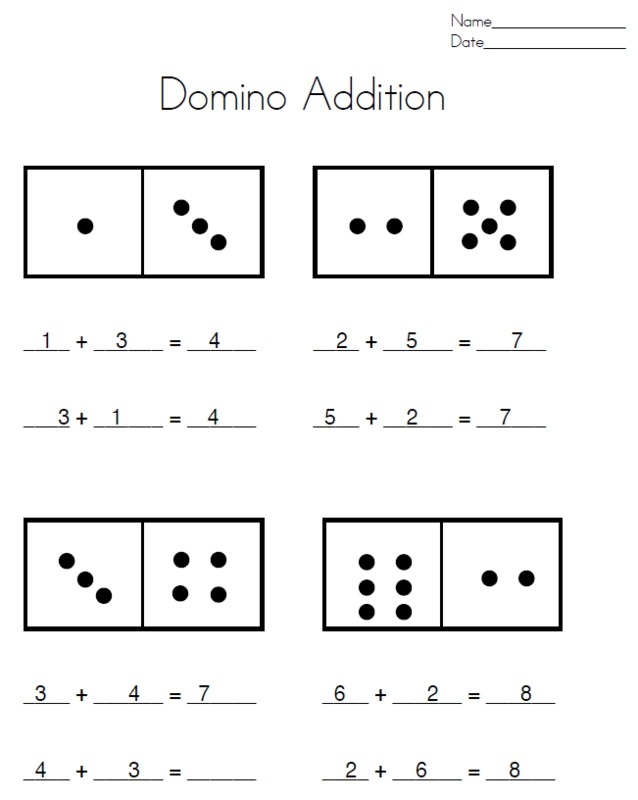##### Engage your students with effective distance learning resources. ACCESS RESOURCES>>

Alignments to Content Standards: 1.OA.B.3

#### Materials

• A large set of dominoes to affix to a whiteboard or place in a pocket chart, or a regular set to use on a document projector.
• One set of dominoes for each student or pair of students

#### Actions

• The teacher asks a child to choose a domino from a stack or bag. As the teacher holds up the domino, the students call out how many dots are on the domino altogether.
• Next the class counts the number of dots on each end of the domino to check their responses. Then the class names an addition equation that represents the relation between total number of dots and the number of dots on each end. For example, if the domino has 4 dots on one side and 2 dots on the other, the teacher can show the domino with the 4 on the left and the 2 on the right and the class names the equation 4+2=6. The teacher then writes the equation.
• Then the teacher rotates the domino so the 2 is on the left and the 4 is on the right, and the class can name the equation 2+4=6. The teacher then writes the equation. The teacher then draws the dots from the chosen domino on a blank domino.
• Once the students understand the task, they can work on their own. Students should have a set of dominoes to explore individually or with a partner, along with the domino addition worksheet. There are two variants of this task.
1. Students can choose dominoes at random, draw the dot pattern, and write the two related equations.
2. Students can find all of the dominoes that have a particular sum, and then draw all the related dot patterns and equations. For example, they could look for all the dominoes that have 6 dots all together, then draw the dot patterns for those dominoes and write the corresponding equations.

## IM Commentary

The purpose of this task is to help students understand the commutative property of addition. Because the total number of dots is the same regardless of how a domino is oriented, the domino reinforces the idea that the addends can be written in any order. Using dominoes in this way can help bridge between using moveable manipulatives such as cubes and using only symbolic representations of numbers and equations. This task is especially appropriate once students have internalized the dot patterns shown on dominoes and dice. If the teacher does not already have dominoes, they are easily found online and can be printed onto colored construction paper and cut out.

If students are asked to first classify their dominoes by their sums, then a discussion can take place relating to which dominoes represent a new sum by being rotated. In other words, when we rotate the domino with a 3 and 1, we get a different equation, but when we rotate the domino with 2 and 2 we don't. This can introduce or reinforce doubles facts.

For some students, the most challenging portion of this task may be copying the dots in a pattern on their blank domino grids. Since the task is about the commutative property of addition, precise placement of the dots is not essential. However, the students do need to have the correct number of dots on each side to represent the addition equation.

The domino grids presented are for a set of double 6 dominoes. Students who are more advanced in addition can be given a set of double 9 dominoes to work with and can also draw their own dominoes as part of their solutions.

This is an activity that can precede Fact Family Houses, where subtraction is included as one of the types of equations.

The Standards for Mathematical Practice focus on the nature of the learning experiences by attending to the thinking processes and habits of mind that students need to develop in order to attain a deep and flexible understanding of mathematics. Certain tasks lend themselves to the demonstration of specific practices by students. The practices that are observable during exploration of a task depend on how instruction unfolds in the classroom. While it is possible that tasks may be connected to several practices, only one practice connection will be discussed in depth. Possible secondary practice connections may be discussed but not in the same degree of detail.

This particular task helps illustrate Mathematical Practice Standard 8, Look for and express regularity in repeated reasoning. First graders might notice a pattern as they create equations for the dominos that there is a relationship between the total numbers of dots and the number of dots on each end. They observe that when the dots on each end are reversed the equation is different but the sum is the same. For instance 3 dots + 4 dots = 7 dots but 4 dots + 3 dots = 7 dots also. In addition, students may notice that if there are the same number of dots at both ends of the domino, only one addition equation is possible. They may wonder, “Are these observations always true?” When students are engaged in MP.8, they purposely look for these types of patterns, make conjectures about these patterns, consider generalities and limitations, and make connections over time about their ideas.  As they begin to explain why these generalizations must be true, they construct, critique, and compare arguments (MP.3).

## Attached Resources

• Domino Addition (any number) Printable
• ## Solution